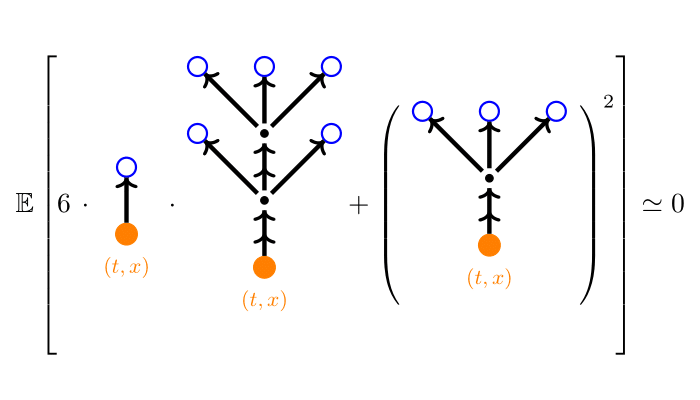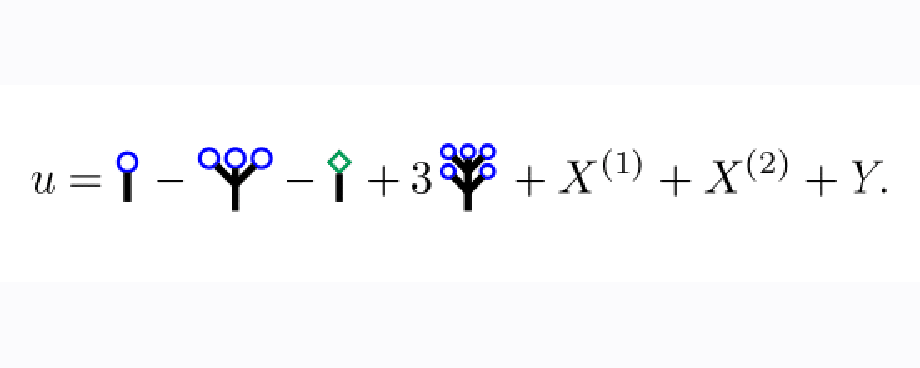### Highlights#### Gibbs measures and nonlinear wave equations

ICERM - August 2022image.png 8.04 KBGibbs measures are tools from probability that play a fundamental role in mathematics (partial differential equations (PDE), stochastic partial differential equations, etc.) and in physics (statistical mechanics, Euclidean field theories, etc). They are written formally

$$d \mu (\phi) = \frac{1}{Z(\beta)} \exp(- \beta H(\phi))$$

where H(·) is "the energy" associated to a given system (e.g.~a Hamiltonian PDE) and we may regard (φ) as the probability of the system to be in state φ. Such a measure can be formally seen to be invariant under the dynamics of the system. For infinite dimensional systems (e.g.~PDE) the construction of a (nontrivial) probability measure and the rigorous justification of its invariance are challenging problems.

One of the most ubiquitous Hamiltonian PDE is the nonlinear wave equation. The recent paper Invariant Gibbs measures for the three dimensional cubic nonlinear wave equation,  by Bjoern Bringmann, Yu Deng, Andrea R. Nahmod and Haitian Yue, available at arXiv:2205.03893, resolves the problem of establishing that the three dimensional Gibbs measure is invariant under the flow of the cubic nonlinear wave equation, and proves that its solutions are globally well-posed for almost all initial conditions. This is the hyperbolic counterpart to the result establishing the same for the parabolic dynamical $$\Phi^4_3$$-model (by Hairer and Hairer-Matetski ).

From a physical perspective, the significance of this work stems from its connections to quantum field theory. In fact, the Gibbs measure is essentially identical to the $$\Phi^4_3$$-measure, whose construction was one of the crowning achievements of the constructive quantum field theory program (e.g.~work of Glimm-Jaffe). From a mathematical perspective, the interest in the main theorem and its proof stems from connections to several areas of mathematical research. At its core, the theorem concerns a refined understanding of how randomness gets transported by the flow of a nonlinear equation which involves probability theory and partial differential equations. The proof utilizes methods from combinatorics, elementary geometry, harmonic analysis, random matrix theory, and nonlinear Fourier analysis.

This work was done in part while the four authors were in residence at ICERM in Fall 2021 for the semester program Hamiltonian Methods in Dispersive and Wave Evolution Equations